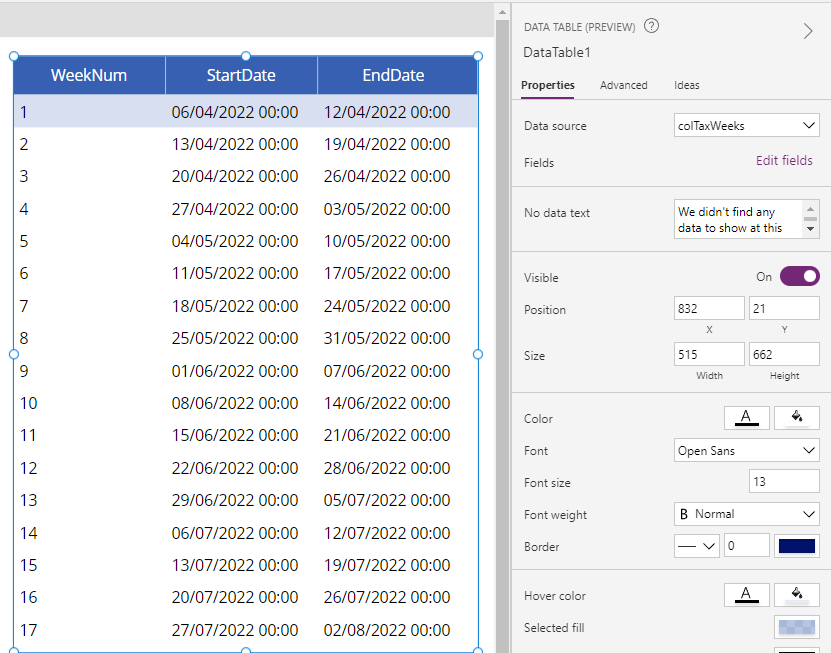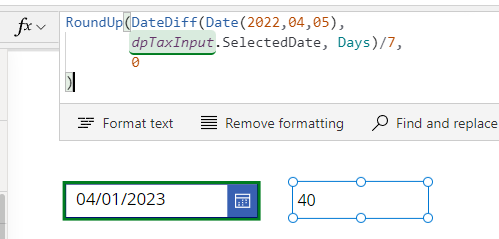# Dates - How to calculate UK tax weeks

If you need to convert dates to UK tax weeks or generate a list of the start and end dates for tax weeks in a year, this post describes some formula that you can use.

When building financial-type apps, there may be a need to work with UK tax weeks. This post describes how UK tax weeks work, and presents some formula that can help us with this task.

## How do UK tax weeks and numbers work?

The HMRC site best describes how UK tax weeks and numbers work.

https://www.gov.uk/government/publications/cwg2-further-guide-to-paye-and-national-insurance-contributions/2022-to-2023-employer-further-guide-to-paye-and-national-insurance-contributions

Income Tax weeks (tax weeks) are periods of 7 days which follow on from each other starting on 6 April each year. The first tax week is 6 April to 12 April inclusive, the second tax week is 13 April to 19 April inclusive, and so on.

The odd day or days at the end of the last complete tax week in the year, (5 April or in leap years, 4 April and 5 April) are treated as a whole tax week, which is tax week 53.
Income Tax months (tax months)

Income Tax months are periods following on from each other in an Income Tax year. They start on the sixth of one month and finish on the fifth of the following month. The first Income Tax month is 6 April to 5 May inclusive, the second Income Tax month is 6 May to 5 June inclusive, and so on.

An easy way to visualise the above is through a tax calendar, such as the one from Sage shown below.

## How to generate a list of the start and end dates for UK tax weeks

Effectively, UK tax weeks always start on the 6th April. Week 2 starts 7 days after 6th April, and so on until the 5th April for the following year.

Therefore, we can generate the start and end dates for the tax weeks in a year by creating a sequence of 53 sequential numbers. We iterate through the sequence with ForAll, and calculate the start and end dates based on a calculation of multiplying the sequence number by 7.

Here is the formula to create a list of UK tax weeks for the financial year 2022.

`ClearCollect(    colTax,    With(        {            startDate: Date(2022, 4, 6)        },        ForAll(            Sequence(53),            {                WeekNum: Value,                StartDate: DateAdd(                    startDate,                    (Value - 1) * 7                ),                EndDate: Min(                          DateAdd(                              startDate,                              (Value * 7) - 1                          ),                          DateAdd(                             DateAdd(startDate, 1, Years),                             -1, Days                         )               )            }        )    ))`
The above formula collects the tax weeks into a collection called colTax. Here's how the result looks when we display the result in a data table.An important point is week 53. This will contain 1 or 2 days, depending on whether the week is a leap year. Our calculation takes account of this by using the Min function to ensure that the final end date can never be greater than the start date (6th April) plus 1 year.

To verify that the result is correct, we can sort the data table in descending sequence and we can see that the end date for week 53 correctly ends on 5th April.## How to get the UK tax week number for an input date (based on a specific tax year)

Another common requirement is to take an input date and calculate the UK tax week number corresponding to the input date.

Since UK tax weeks always begin on 6th April, we can make this calculation by working out the number of days that have elapsed since 6th April. We then work out the number of weeks by dividing by 7 and that will give us the tax week number. Since the first week is week 1, we must round up to the next highest number.

The formula to derive the week number for the tax year 2022-2023 looks like this:

`RoundUp(DateDiff(Date(2022,04,05),         dpTaxInput.SelectedDate, Days)/7,         0)`
In this example, the input date comes from a date picker control called dpTaxInput. The screenshot below shows how the formula returns the expected result for the input date 4th January 2023 (week 40).## How to get the UK tax week number from an input date (generic formula)

The initial formula above highlights the principle of how to calculate the tax week for an input date. However, the formula is hard-coded to use 5th April 2022 (ie - the UK tax year 2022-2023).

A more generic formula that avoids the need to specify the tax year looks like this. This includes the conditional logic that's required to calculate the base tax year. For example, an input date of 7th March 2023 will correspond to the tax year starting 2022 because the input date is less than 6th April of the input year.

`With({inputDate:dpTaxInput.SelectedDate},     With({taxYearStart:        If(Month(inputDate) >=4 && Day(inputDate) >5,         Date(Year(inputDate),04,05),        Date(Year(inputDate)-1,04,05))     },      RoundUp(DateDiff(taxYearStart,                     inputDate,Days)/7, 0       )    ))`

## Conclusion

This post demonstrated formula to calculate the UK tax week for a given input date and to generate a list of the start and end dates for tax weeks in a year, These types of calculations can be very useful when building financial type apps.